# Electronics and Communication Engineering - Electromagnetic Field Theory

1.
For an incident wave which is normal on the perfect dielectric the reflection factor is given by (where ε1 and ε2 are the permittivities of 1st and 2nd medium respectively)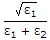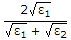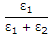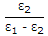Explanation:
No answer description is available. Let's discuss.

2.
For EM wave propagation in free space, the free space is defined as
σ = 0, ε = 1, μ ≠ 1,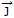= 0
σ = 0, ε = 1, μ = 1,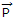= 0,= 0
σ ≠ 0, ε > 1, μ < 1,≠ 0,= 0
σ = 0, ε = 1, μ = 1,≠ 0,≠ 0
Explanation:
No answer description is available. Let's discuss.

3.
For TE or TM modes of propagation in bounded media, the phase velocity is
independent of frequency
is a linear function of frequency
is a non-linear function of frequency
can be frequency dependent or frequency independent on the source
Explanation:
No answer description is available. Let's discuss.

4.
Frequencies in the UHF range propagate by means of
space wave
surface waves
sky waves
ground waves